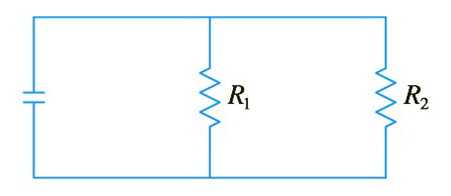Chapter 2.8, Problem 39E

Chapter
Section
Textbook Problem

# If two resistors with resistances R 1  and  R 2 are connected in parallel, as in the figure, then the total resistance R, measured in ohms ( Ω ) , is given by 1 R = 1 R 1 + 1 R 2 If R 1  and  R 2 are increasing at rates of 0.3 Ω / s and  0.2 Ω / s , respectively, how fast is R changing when R 1 = 80 Ω  and  R 2 = 100 Ω ?To determine

To find:

The rate at which R is changing.

Explanation

1. Concept:

Using parallel resistors with total resistance formula and differentiation, we can find the rate of change.

2. Formula:

i. Total resistance: 1R=1R1 +1R2

ii. Power rule of differentiation: ddxxn=n*xn-1

iii. Chain rule of differentiation: ddtfx=ddxfx*dxdt

3. Given:

i. dR1dt=0.3

ii. dR2dt=0.2

iii. R1=80 and R2=100

4. Calculations:

Consider the formula of total resistance 1R=1R1 +1R2

Substituting the values

1R=180+1100 =>

### Still sussing out bartleby?

Check out a sample textbook solution.

See a sample solution

#### The Solution to Your Study Problems

Bartleby provides explanations to thousands of textbook problems written by our experts, many with advanced degrees!

Get Started

#### Convert the expressions in Exercises 6584 to power form. xy23

Finite Mathematics and Applied Calculus (MindTap Course List)

#### In Exercises 4144, determine whether the statement is true or false. 44. 561112

Applied Calculus for the Managerial, Life, and Social Sciences: A Brief Approach

#### limx6x33+x= a) b) c) 0 d) 2

Study Guide for Stewart's Single Variable Calculus: Early Transcendentals, 8th

#### For

Study Guide for Stewart's Multivariable Calculus, 8th

#### In Exercises 116, determine whether the argument is valid. p~qp_q~q

Finite Mathematics for the Managerial, Life, and Social Sciences